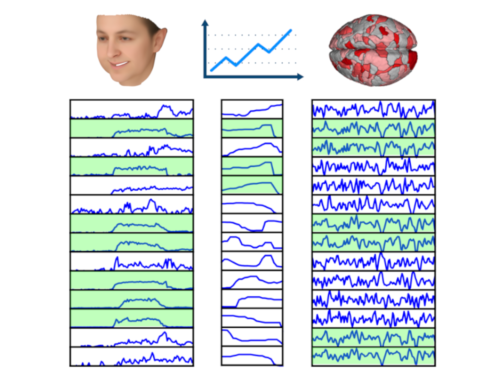•In CVPR’20 presented a new method (with code) for finding the largest subset of synchronized time series from a given set of time series. Specifically, we aim to find the largest subset of time series such that all pairs of in the subset are correlated at least by a (given) threshold value $\rho$. This is an NP-hard problem and the exact solution is, in general, unfeasible. We propose a new method, called SyncRef, for finding an approximate solution in an efficient…